Dishashree26 Gupta — Published On May 21, 2017 and Last Modified On June 25th, 2019

Artificial Intelligence, deep learning, machine learning — whatever you’re doing if you don’t understand it — learn it. Because otherwise you’re going to be a dinosaur within 3 years.

-Mark Cuban

This statement from Mark Cuban might sound drastic – but its message is spot on! We are in the middle of a revolution – a revolution caused by Big Huge data and a ton of computational power.

For a minute, think how a person would feel in early 20th century if he / she did not understand electricity. You would have been used to doing things in a particular manner for ages and all of a sudden things around you started changing. Things which required many people can now be done with one person and electricity. We are going through a similar journey with machine learning & deep learning today.

So, if you haven’t explored or understood the power of deep learning – you should start it today. I have written this article to help you understand common terms used in deep learning.

If you are wondering why I am writing this article – I am writing it because I want you to start your deep learning journey without hassle or without getting intimidated. When I first began reading about deep learning, there were several terms I had heard about, but it was intimidating when I tried to understand them. There are several words which are recurring when we start reading about any deep learning application.

In this article, I have created something like a deep learning dictionary for you which you can refer whenever you need the basic definition of the most common terms used. I hope after this article these terms wouldn’t haunt you anymore.

## Terms related to topics:

To help you understand various terms, I have broken them in 3 different groups. If you are looking for a specific term, you can skip to that section. If you are new to the domain, I would recommend that you go through them in the order I have written them.

1. Basics of Neural Networks
• Common Activation Functions
2. Convolutional Neural Networks
3. Recurrent Neural Networks

## Basics of Neural Networks

1) Neuron- Just like a neuron forms the basic element of our brain, a neuron forms the basic structure of a neural network. Just think of what we do when we get new information. When we get the information, we process it and then we generate an output. Similarly, in case of a neural network, a neuron receives an input, processes it and generates an output which is either sent to other neurons for further processing or it is the final output.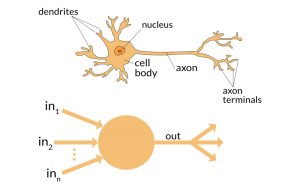2) Weights – When input enters the neuron, it is multiplied by a weight. For example, if a neuron has two inputs, then each input will have has an associated weight assigned to it. We initialize the weights randomly and these weights are updated during the model training process. The neural network after training assigns a higher weight to the input it considers more important as compared to the ones which are considered less important. A weight of zero denotes that the particular feature is insignificant.

Let’s assume the input to be a, and the weight associated to be W1. Then after passing through the node the input becomes a*W1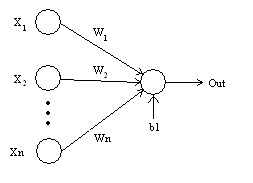3) Bias – In addition to the weights, another linear component is applied to the input, called as the bias. It is added to the result of weight multiplication to the input. The bias is basically added to change the range of the weight multiplied input. After adding the bias, the result would look like a*W1+bias. This is the final linear component of the input transformation.

4) Activation Function – Once the linear component is applied to the input, a non-linear function is applied to it. This is done by applying the activation function to the linear combination.The activation function translates the input signals to output signals. The output after application of the activation function would look something like f(a*W1+b) where f() is the activation function.

In the below diagram we have “n” inputs given as X1 to Xn and corresponding weights Wk1 to Wkn. We have a bias given as bk. The weights are first multiplied to its corresponding input and are then added together along with the bias. Let this be called as u.

u=∑w*x+b

The activation function is applied to u i.e. f(u) and we receive the final output from the neuron as yk = f(u)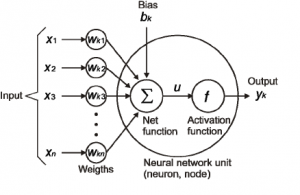### Commonly applied Activation Functions

The most commonly applied activation functions are – Sigmoid, ReLU and softmax

a) Sigmoid – One of the most common activation functions used is Sigmoid. It is defined as:

`sigmoid(x) = 1/(1+e-x)`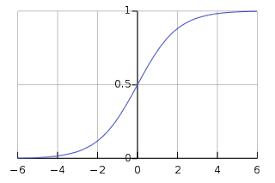###### Source: Wikipedia

The sigmoid transformation generates a more smooth range of values between 0 and 1. We might need to observe the changes in the output with slight changes in the input values. Smooth curves allow us to do that and are hence preferred over step functions.

b) ReLU(Rectified Linear Units) – Instead of sigmoids, the recent networks prefer using ReLu activation functions for the hidden layers. The function is defined as:

`f(x) = max(x,0).`

The output of the function is X when X>0 and 0 for X<=0. The function looks like this: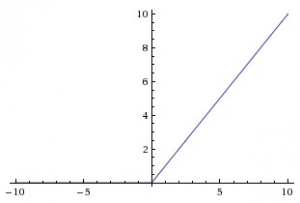###### source: cs231n

The major benefit of using ReLU is that it has a constant derivative value for all inputs greater than 0. The constant derivative value helps the network to train faster.

c) Softmax – Softmax activation functions are normally used in the output layer for classification problems. It is similar to the sigmoid function, with the only difference being that the outputs are normalized to sum up to 1. The sigmoid function would work in case we have a binary output, however in case we have a multiclass classification problem, softmax makes it really easy to assign values to each class which can be easily interpreted as probabilities.

It’s very easy to see it this way – Suppose you’re trying to identify a 6 which might also look a bit like 8. The function would assign values to each number as below. We can easily see that the highest probability is assigned to 6, with the next highest assigned to 8 and so on…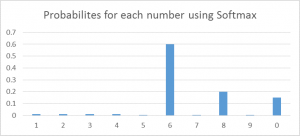5) Neural Network – Neural Networks form the backbone of deep learning.The goal of a neural network is to find an approximation of an unknown function. It is formed by interconnected neurons. These neurons have weights, and bias which is updated during the network training depending upon the error. The activation function puts a nonlinear transformation to the linear combination which then generates the output. The combinations of the activated neurons give the output.

A neural network is best defined by “Liping Yang” as –

Neural networks are made up of numerous interconnected conceptualized artificial neurons, which pass data between themselves, and which have associated weights which are tuned based upon the network’s “experience.” Neurons have activation thresholds which, if met by a combination of their associated weights and data passed to them, are fired; combinations of fired neurons result in “learning”.

6) Input / Output / Hidden Layer – Simply as the name suggests the input layer is the one which receives the input and is essentially the first layer of the network. The output layer is the one which generates the output or is the final layer of the network. The processing layers are the hidden layers within the network. These hidden layers are the ones which perform specific tasks on the incoming data and pass on the output generated by them to the next layer. The input and output layers are the ones visible to us, while are the intermediate layers are hidden.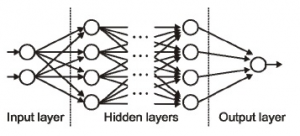###### Source: cs231n

7) MLP (Multi Layer perceptron) – A single neuron would not be able to perform highly complex tasks. Therefore, we use stacks of neurons to generate the desired outputs. In the simplest network we would have an input layer, a hidden layer and an output layer. Each layer has multiple neurons and all the neurons in each layer are connected to all the neurons in the next layer. These networks can also be called as fully connected networks.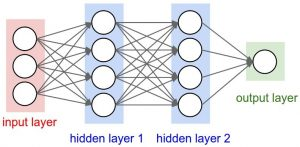8) Forward Propagation – Forward Propagation refers to the movement of the input through the hidden layers to the output layers. In forward propagation, the information travels in a single direction FORWARD. The input layer supplies the input to the hidden layers and then the output is generated. There is no backward movement.

9) Cost Function – When we build a network, the network tries to predict the output as close as possible to the actual value. We measure this accuracy of the network using the cost/loss function. The cost or loss function tries to penalize the network when it makes errors.

Our objective while running the network is to increase our prediction accuracy and to reduce the error, hence minimizing the cost function. The most optimized output is the one with least value of the cost or loss function.

If I define the cost function to be the mean squared error, it can be written as –

C= 1/m ∑(y – a)2 where m is the number of training inputs, a is the predicted value and y is the actual value of that particular example.

The learning process revolves around minimizing the cost.

10) Gradient Descent – Gradient descent is an optimization algorithm for minimizing the cost. To think of it intuitively, while climbing down a hill you should take small steps and walk down instead of just jumping down at once. Therefore, what we do is, if we start from a point x, we move down a little i.e. delta h, and update our position to x-delta h and we keep doing the same till we reach the bottom. Consider bottom to be the minimum cost point.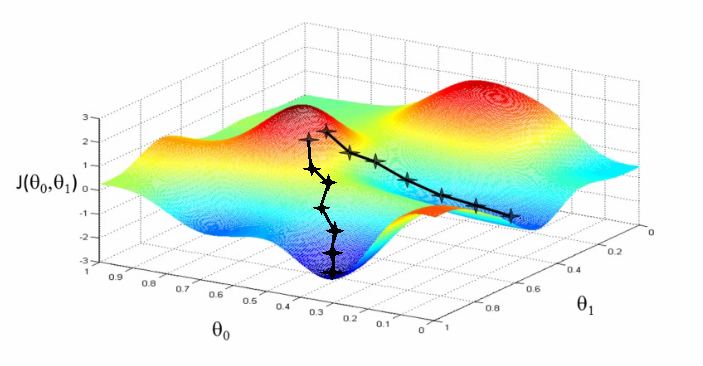Source

Mathematically, to find the local minimum of a function one takes steps proportional to the negative of the gradient of the function.

11) Learning Rate – The learning rate is defined as the amount of minimization in the cost function in each iteration. In simple terms, the rate at which we descend towards the minima of the cost function is the learning rate. We should choose the learning rate very carefully since it should neither be very large that the optimal solution is missed and nor should be very low that it takes forever for the network to converge.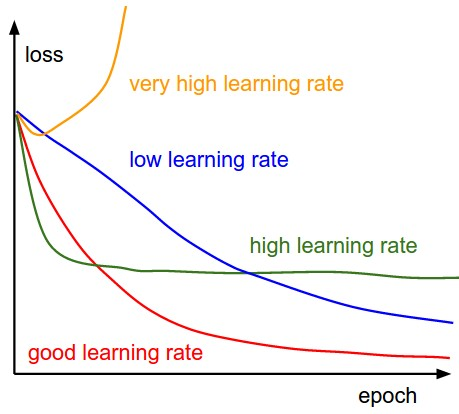Source

12) Backpropagation – When we define a neural network, we assign random weights and bias values to our nodes. Once we have received the output for a single iteration, we can calculate the error of the network. This error is then fed back to the network along with the gradient of the cost function to update the weights of the network. These weights are then updated so that the errors in the subsequent iterations is reduced. This updating of weights using the gradient of the cost function is known as back-propagation.

In back-propagation the movement of the network is backwards, the error along with the gradient flows back from the out layer through the hidden layers and the weights are updated.

13) Batches – While training a neural network, instead of sending the entire input in one go, we divide in input into several chunks of equal size randomly. Training the data on batches makes the model more generalized as compared to the model built when the entire data set is fed to the network in one go.

14) Epochs – An epoch is defined as a single training iteration of all batches in both forward and back propagation. This means 1 epoch is a single forward and backward pass of the entire input data.

The number of epochs you would use to train your network can be chosen by you. It’s highly likely that more number of epochs would show higher accuracy of the network, however, it would also take longer for the network to converge. Also you must take care that if the number of epochs are too high, the network might be over-fit.

15) Dropout – Dropout is a regularization technique which prevents over-fitting of the network. As the name suggests, during training a certain number of neurons in the hidden layer is randomly dropped. This means that the training happens on several architectures of the neural network on different combinations of the neurons. You can think of drop out as an ensemble technique, where the output of multiple networks is then used to produce the final output.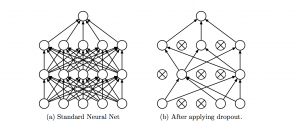###### Source: Original paper

16) Batch Normalization – As a concept, batch normalization can be considered as a dam we have set as specific checkpoints in a river. This is done to ensure that distribution of data is the same as the next layer hoped to get. When we are training the neural network, the weights are changed after each step of gradient descent. This changes the how the shape of data is sent to the next layer.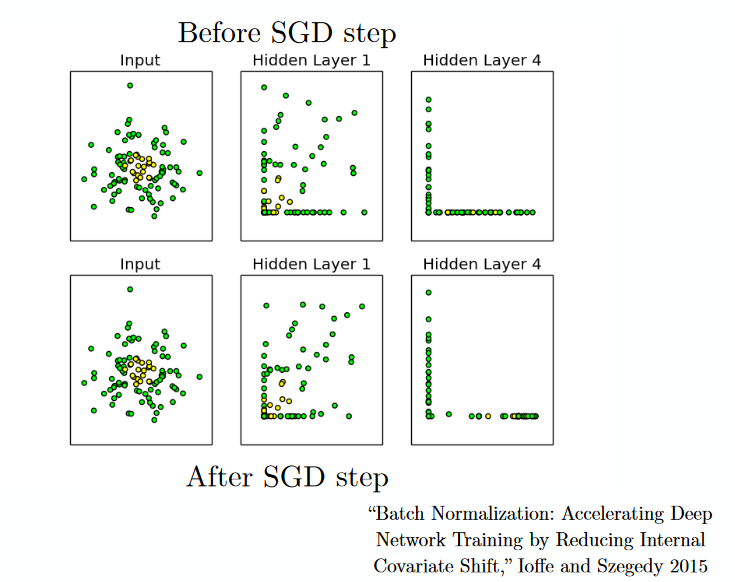But the next layer was expecting the distribution similar to what it had previously seen. So we explicitly normalize the data before sending it to the next layer.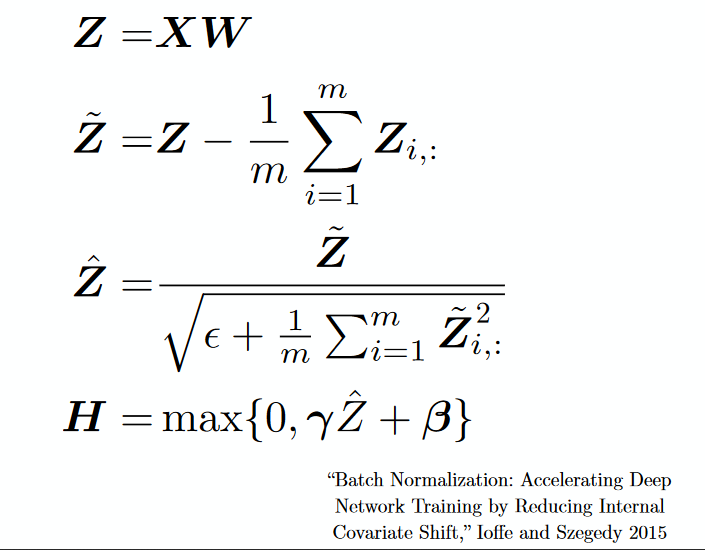## Convolutional Neural Networks

17) Filters – A filter in a CNN is like a weight matrix with which we multiply a part of the input image to generate a convoluted output. Let’s assume we have an image of size 28*28. We randomly assign a filter of size 3*3, which is then multiplied with different 3*3 sections of the image to form what is known as a convoluted output. The filter size is generally smaller than the original image size. The filter values are updated like weight values during backpropagation for cost minimization.

Consider the below image. Here filter is a 3*3 matrix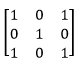which is multiplied with each 3*3 section of the image to form the convolved feature.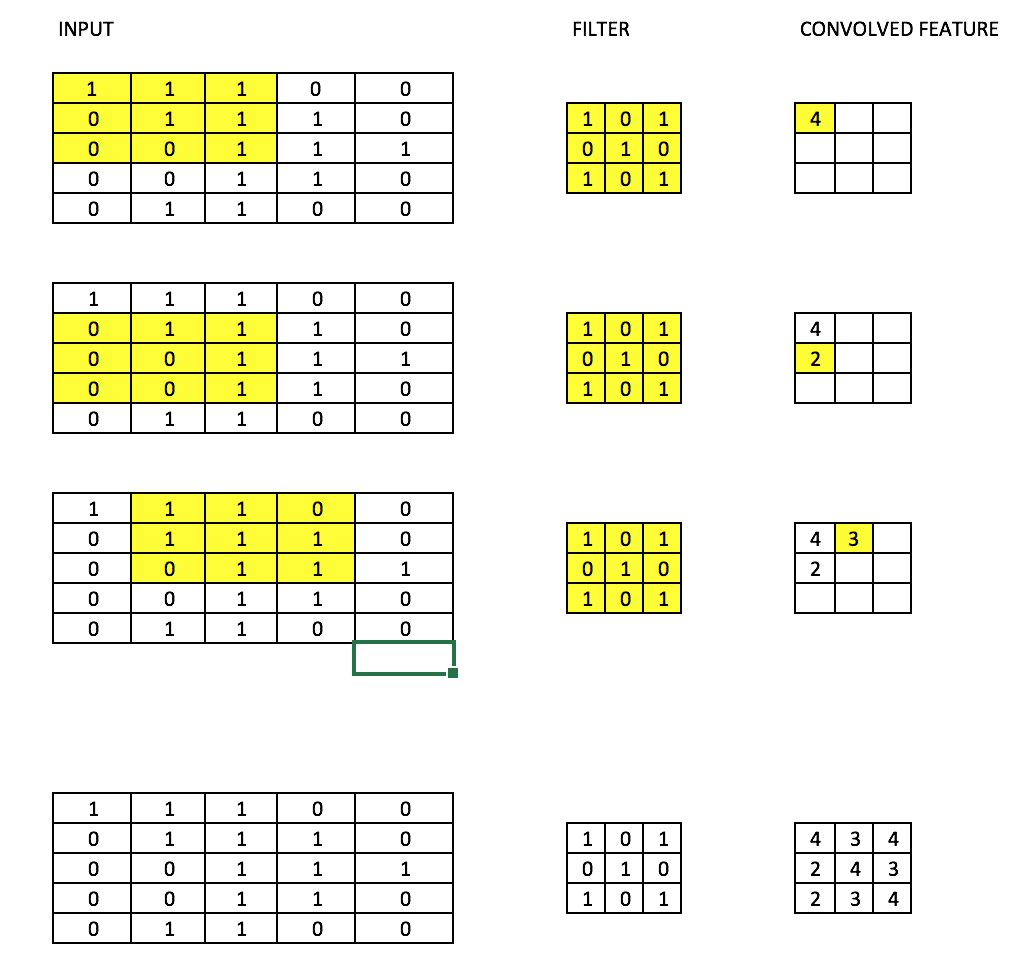18) CNN (Convolutional neural network) – Convolutional neural networks are basically applied on image data. Suppose we have an input of size (28*28*3), If we use a normal neural network, there would be 2352(28*28*3) parameters. And as the size of the image increases the number of parameters becomes very large. We “convolve” the images to reduce the number of parameters (as shown above in filter definition). As we slide the filter over the width and height of the input volume we will produce a 2-dimensional activation map that gives the output of that filter at every position. We will stack these activation maps along the depth dimension and produce the output volume.

You can see the below diagram for a clearer picture.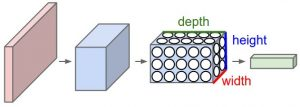###### Source: cs231n

19) Pooling – It is common to periodically introduce pooling layers in between the convolution layers. This is basically done to reduce a number of parameters and prevent over-fitting. The most common type of pooling is a pooling layer of filter size(2,2) using the MAX operation. What it would do is, it would take the maximum of each 4*4 matrix of the original image.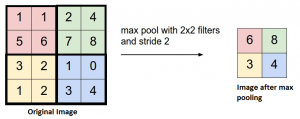###### Source: cs231n

You can also pool using other operations like Average pooling, but max pooling has shown to work better in practice.

20) Padding – Padding refers to adding extra layer of zeros across the images so that the output image has the same size as the input. This is known as same padding.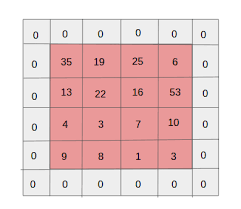After the application of filters  the convolved layer in the case of same padding has the size equal to the actual image.

Valid padding refers to keeping the image as such an having all the pixels of the image which are actual or “valid”. In this case after the application of filters the size of the length and the width of the output keeps getting reduced at each convolutional layer.

21) Data Augmentation – Data Augmentation refers to the addition of new data derived from the given data, which might prove to be beneficial for prediction. For example, it might be easier to view the cat in a dark image if you brighten it, or for instance, a 9 in the digit recognition might be slightly tilted or rotated. In this case, rotation would solve the problem and increase the accuracy of our model. By rotating or brightening we’re improving the quality of our data. This is known as Data augmentation.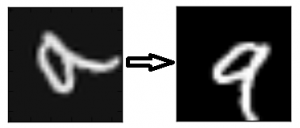## Recurrent Neural Network

22) Recurrent Neuron – A recurrent neuron is one in which the output of the neuron is sent back to it for t time stamps. If you look at the diagram the output is sent back as input t times. The unrolled neuron looks like t different neurons connected together. The basic advantage of this neuron is that it gives a more generalized output.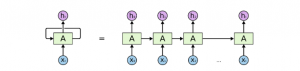###### Source: cs231n

23) RNN(Recurrent Neural Network) – Recurrent neural networks are used especially for sequential data where the previous output is used to predict the next one. In this case the networks have loops within them. The loops within the hidden neuron gives them the capability to store information about the previous words for some time to be able to predict the output. The output of the hidden layer is sent again to the hidden layer for t time stamps. The unfolded neuron looks like the above diagram. The output of the recurrent neuron goes to the next layer only after completing all the time stamps. The output sent is more generalized and the previous information is retained for a longer period.

The error is then back propagated according to the unfolded network to update the weights. This is known as backpropagation through time(BPTT).

24) Vanishing Gradient Problem – Vanishing gradient problem arises in cases where the gradient of the activation function is very small. During back propagation when the weights are multiplied with these low gradients, they tend to become very small and “vanish” as they go further deep in the network. This makes the neural network to forget the long range dependency. This generally becomes a problem in cases of recurrent neural networks where long term dependencies are very important for the network to remember.

This can be solved by using activation functions like ReLu which do not have small gradients.

25) Exploding Gradient Problem – This is the exact opposite of the vanishing gradient problem, where the gradient of the activation function is too large. During back propagation, it makes the weight of a particular node very high with respect to the others rendering them insignificant. This can be easily solved by clipping the gradient so that it doesn’t exceed a certain value.

## End Notes

I hope you enjoyed going through the article. I have given a high level overview of the basic deep learning terms. I hope you now have a basic understanding of these terms. I have tried to explain everything in a language as easy as possible, however in case of any doubts/clarifications, please feel free to drop in your comments.

### Learn, compete, hack and get hired!###### Dishashree26 Gupta

Dishashree is passionate about statistics and is a machine learning enthusiast. She has an experience of 1.5 years of Market Research using R, advanced Excel, Azure ML.

## 38 thoughts on "25 Must Know Terms & concepts for Beginners in Deep Learning"###### Arun says:May 22, 2017 at 6:01 am
Thanks for the article . Nice Work and a good one for beginners !! Reply###### Dr Venugopala Rao says:May 22, 2017 at 6:25 am###### Deepthi says:May 22, 2017 at 6:32 am
The imformation and approach given for Neural network is really explicit. Reply###### Gaurav says:May 22, 2017 at 7:21 am
Another great article where a complex topic is dissected into smaller and simpler topics. Waiting for more such articles. Reply###### Kishore says:May 22, 2017 at 9:27 am###### Surya says:May 22, 2017 at 10:48 am
Well explained...However I would like to know the difference between Convolution layer and pooling layers. Reply###### Allison says:May 22, 2017 at 2:07 pm
Great article, very clear and simplified. Thank you. Reply###### V Reddy says:May 22, 2017 at 2:43 pm###### NaveenHN says:May 22, 2017 at 4:09 pm###### Eduardo says:May 23, 2017 at 12:27 am
Thanks for the article. I would like to see a code in C# or python 2.7 for single layer perceptron. Reply###### Abhinav Goel says:May 23, 2017 at 4:44 am
This is superbly written and easy to understand! Kudos! Reply###### Dishashree Gupta says:May 23, 2017 at 6:05 am
Hi Surya, Convolution layer is the basic building block of CNN. It is the one which is obtained by applying filters. Pooling layers however are just applied for feature reduction and reduce overfitting. We just apply the max function over defined strides of input data. Reply###### Top 10 Engineering College in India says:May 23, 2017 at 10:14 am
Thank you for sharing such a great & knowledgeable blog. Reply###### Keith S. Safford says:May 23, 2017 at 12:02 pm
Excellent description of key concepts and overview of Deep Learning. Am just getting into this after 32 years in IT as I have always enjoyed statistics and started learning R and will be teaching Python in the fall (University where I am an adjunct faculty member is switching from VB.NET to Python). Reply###### Dishashree Gupta says:May 23, 2017 at 8:11 pm###### Marc Nolan says:May 23, 2017 at 2:41 pm
Excellent overview and very concise. I look forward to your added documents and "deeper" discussions around this industry. Reply###### Avi says:May 23, 2017 at 2:52 pm
Good article. Made it easy to understand such complicated stuff. Thanks to the Author :) Reply###### abhishek bisht says:May 23, 2017 at 5:40 pm
very useful article, learn a lot, thanks Reply###### Dishashree Gupta says:May 23, 2017 at 8:00 pm###### Dishashree Gupta says:May 23, 2017 at 8:09 pm
Hope Analytics Vidhya helps you ! Reply###### Dishashree Gupta says:May 23, 2017 at 8:10 pm
Much more is there in the pipeline ! Reply###### Kiran says:May 24, 2017 at 4:11 pm
This is awesome ! All aspects are well described. Thank you. Reply###### Nitin Mahajan says:May 26, 2017 at 7:10 am
Super Awesome Explanation! Thank you for the wonderful article . Cannot get better than this. Reply###### Aman Thakur says:June 10, 2017 at 4:24 pm
Very useful post thanks for sharing this. Reply###### Sai Raghuram Kothapalli says:July 01, 2017 at 8:17 am
Always loved such sweet and simple articles. Thank you so much for this info. Reply###### Yvette says:July 05, 2017 at 10:09 pm###### Amit says:July 25, 2017 at 5:37 pm
Great.. Lot of terms are there. This would be helpful for beginners like me when reading any article on Deep Learning. Reply###### karbhari kale says:July 29, 2017 at 5:31 pm###### Amr Abo Eid says:August 17, 2017 at 9:51 am
what the next step to be able for creating model ? Reply###### Rebeen says:August 17, 2017 at 8:20 pm
Thank you very much thanks for sharing this, please could you put an example of Transfer learning for text data. thanks Reply###### Faiz says:September 28, 2017 at 5:46 pm
Thanks Dipashree for the article .this is indeed deep learning networks explained in most lucid form. Reply###### samah says:November 06, 2017 at 2:22 pm
Great job thank you. about the batch normalisation; is-it done after each epoch and for each layer? what represente the Z and the H in the normalisation function? Reply###### May says:November 09, 2017 at 7:24 am
Amazing introduction. I'm starting to learn neural networks for a research project, and I learned a lot from this article. :) Thanks! Reply###### Rashmi G says:January 09, 2018 at 4:44 pm
Great article both as a refresher for experts and a good starting point vor freshers like me..Thank you.I learned a lot from this... Reply###### Pradeep kumar says:March 22, 2018 at 6:45 pm